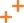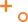# 生成随机数的方式你选对了吗？

ID：shouwangxiansheng

## rand

rand函数声明如下：

#include <stdlib.h>
int rand(void);


​rand函数返回[0,RAND_MAX）范围的随机整数，在我的机器上，RAND_MAX为2147483647。

/*来源：公众号【编程珠玑】
rand.c
*/
#include<stdlib.h>
#include<stdio.h>
int main(void)
{
int i = 0;
while(i < 5)
{
printf("%d ",rand());
i++;
}
printf("\n");
return 0;
}


\$ gcc -o rand rand.c
./rand
1804289383 846930886 1681692777 1714636915 1957747793


## srand

#include <stdlib.h>
void srand(unsigned int seed);


/*来源：公众号【编程珠玑】
rand.c
*/
#include<stdlib.h>
#include<stdio.h>
#include<time.h>
int main(void)
{
srand(time(NULL));//设置随机种子，注意只需要设置一次即可
int i = 0;
while(i < 5)//生成5个随机数
{
printf("%d ",rand());
i++;
}
printf("\n");
return 0;
}


## rand_r

#include <stdlib.h>
int rand_r(unsigned int *seedp);


//来源：公众号【编程珠玑】
#include<stdlib.h>
#include<stdio.h>
#include<time.h>
int main(void)
{
unsigned int seed = time(NULL);
int i = 0;
while(i < 5)//生成5个随机数
{
printf("%d ",rand_r(&seed));
i++;
}
printf("\n");
return 0;
}


unsigned int seed  = time(NULL)^pthread_self();


## random

#include <stdlib.h>
long int random(void);
void srandom(unsigned int seed);


random返回的类型为long int，因此在一定程度上，它生成的范围要大得多。另外与rand类似，需要使用srandom函数设置种子。具体的例子就不再放出了。

## 生成指定范围随机数

[a,b)

 (rand() % (b - a)) + a;


[a,b]

 (rand() % (b - a + 1)) + a;


(a,b]

(rand() % (b-a)) + a + 1;


[0,b]

rand() % b ;


0到1之间的浮点数

rand()/(double)RAND_MAX;


//来源：公众号【编程珠玑】
#include<stdlib.h>
#include<stdio.h>
#include<time.h>
int main(void)
{
srand(time(NULL));//设置随机种子，注意只需要设置一次即可
int i = 0;
int a = 2;
int b = 10;
while(i < 5)//生成5个随机数
{
printf("%d ",( rand() % ( b - a ) )+ a);
i++;
}
printf("\n");
return 0;
}


## 总结08-31128604-2376
08-18
06-061万+
03-29369
11-29165
01-14808
08-201224
09-051万+
06-043万+